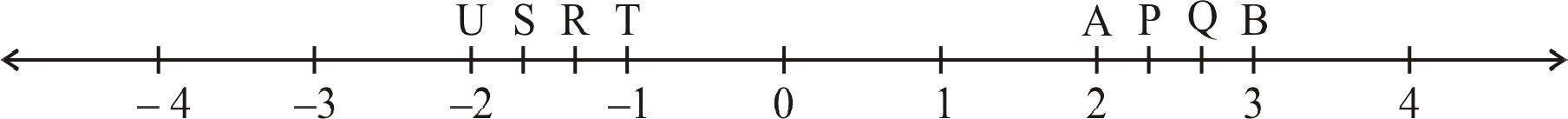# Ex.9.1 Q5 Rational-Numbers Solution - NCERT Maths Class 7

Go back to  'Ex.9.1'

## Question

The points $$P, Q, R, S, T, U, A$$ and $$B$$ on the number line are such that, $$TR = RS = SU$$ and $$AP = PQ = QB$$. Name the rational numbers represented by $$P, Q, R$$ and $$S$$.Video Solution
Rational Numbers
Ex 9.1 | Question 5

## Text Solution

Steps:

Distance between $$U$$ and $$T = 1$$ unit. It is divided into three equal parts

\begin{align}{\rm{TR}} = {\rm{RS}} = {\rm{SU}} = \frac{1}{3}\\{\rm{R}} = - 1 - \frac{1}{3} = \frac{{ - 4}}{3}\\{\rm{S}} = - 1 - \frac{2}{3} = \frac{{ - 5}}{3}\end{align}

Similarly, $$AB = 1$$ unit

It is divided into three equal parts

\begin{align}{\rm{AP}} = {\rm{PQ}} &= {\rm{QB}} = \frac{1}{3}\\{\rm{P}} = 2 + \frac{1}{3} &= \frac{6}{3} + \frac{1}{3} = \frac{7}{3}\\{\rm{Q}} = 2 + \frac{2}{3} &= \frac{6}{3} + \frac{2}{3} = \frac{8}{3}\end{align}

Thus, the rational number $$P, Q, R$$ and $$S$$ are

\begin{align} \frac{7}{3},\frac{8}{3},\frac{{ - 4}}{3}\,\,{\rm{and}}\,\frac{{ - 5}}{3}\end{align}

Learn from the best math teachers and top your exams

• Live one on one classroom and doubt clearing
• Practice worksheets in and after class for conceptual clarity
• Personalized curriculum to keep up with school